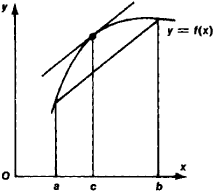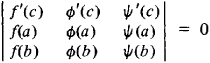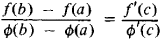# Mean Value Theorem

Also found in: Acronyms, Wikipedia.

## mean value theorem

[′mēn ′val·yü ‚thir·əm]
(mathematics)
The proposition that, if a function ƒ (x) is continuous on the closed interval [a,b ] and differentiable on the open interval (a,b), then there exists x0, a <>x0<>b, such that ƒ(b) - ƒ(a) = (b-a)ƒ′(x0). Also known as first law of the mean; Lagrange's formula; law of the mean.
McGraw-Hill Dictionary of Scientific & Technical Terms, 6E, Copyright © 2003 by The McGraw-Hill Companies, Inc.
The following article is from The Great Soviet Encyclopedia (1979). It might be outdated or ideologically biased.

## Mean Value Theorem

one of the fundamental results of the differential calculus relating an increment of a function f(x) and the values of its derivative. In analytic terms,

f(b) — f(a) = (b — a)f’(c)

where c is some number satisfying the inequality a < c < b. Formula (1) is valid if the function f(x) is continuous on the segment [a, b] and has a derivative at each point of the interval (a, b). In geometric terms (see Figure 1), formula (1) states that the tangent to the curve y = f(x) at a suitable point [c, f(c)] is parallel to the chord passing through the points [a, f(a)] and [b, f(b)]. The mean value theorem was discovered by J. Lagrange in 1797.Figure 1

Among the different generalizations of the mean value theorem, note Bonnet’s mean value formulaand its particular case, Cauchy’s mean value formulaThe Great Soviet Encyclopedia, 3rd Edition (1970-1979). © 2010 The Gale Group, Inc. All rights reserved.
References in periodicals archive ?
Based on Lagrange's mean value theorem, consider the following.
If s [greater than or equal to] k, by the mean value theorem, there exists [eta] [member of] (k,k + [1/k]) such that
For the left hand side of (67), we use the Mean Value Theorem; then there exists number [tau] [member of] [[t.sub.1], [t.sub.2]] such that
By using the mean value theorem and fuzzy logic system (FLS), the parameter updated laws are constructed to estimate the unknown adaptive controller parameters online.
We demonstrate below another example of a traditionally static core, Mean Value or Cauchy Mean Value Theorem, done differently.
Our sequence for teaching the Mean Value Theorem illustrates the SimCalc approach.
(2) In order to handle the nonaffine coupling terms, the implicit function theorem and the mean value theorem are invoked, respectively.
For linear case by using Lemma 1, Theorem 2 and the Integral mean value theorem, we get
By the mean value theorem and (26), for any closed interval contained in t [member of] ([[tau].sub.k], [[tau].sub.k+1]], k = m,m + 1, ..., and [[tau].sub.m] > [??], one has
(The mean value theorem for [nabla]-integrals) If f : J [right arrow] E is [nabla]- integrable then

Site: Follow: Share:
Open / Close